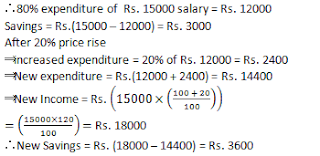### Quant Quiz- Miscellaneous for SSC CGL Mains, SSC CHSL Set-5

Dear Readers, We are providing you a Quant Quiz for  SSC CGL Mains, SSC CHSL based on previous papers.

1. A student finds the average of 10 two digit numbers. If the digits of a number are interchanged, the average increases by 3.6. The difference between the digits of that two digit number is:-
1. 4
2. 3
3. 2
4. 5

2. A shopkeeper allows a discount of 10% on the marked price of an item. But charges a sales tax of 8% on the discounted price. If the customer pays 3402₹ as the price including the sales tax, then the marked price is :-
3400
3500
2600
3800

3. A car travels from city P to city Q at a constant speed. If its speed increased by 10km/h, it would have been taken one hour lesser to cover the same distance. It would have taken further 45 minutes lesser , if the speed was further increased by 10km/h. The distance between the two cities is :-

340
420
600
500

4. First and second numbers are less than a third number by 30% and 37%, respectively. The second number is less than the first by:
7%
4%
3%
10%

5. If the height of a triangle is decreased by 40% and its base is increased by 40%, what will be the effect on its area?
No change
16% increase
8% increase
16% decrease

6. In 1 kg mixture of sand and iron, 20% is iron. How much sand should be added so that the proportion of iron becomes 10%?
1 kg
200 gm
800 mgs
1.8 kg

7. A tradesman gives 4% discount on the marked price and gives 1 article free for buying every 15 articles and thus gains 35%. The marked price is increased above the cost price by:
40%
39%
50%
20%

8. The strength of a school increases and decreases in every alternate year by 10%. It started with increase in 2000. Then the strength of the school in 2003 as compares to that in 2000 was:
Increased by 8.9%
Decreased by 8.9%
Increase by 9.8%
Decreased by 9.8%

9. The price of cooking oil has increased by 25%. The percentage of reduction that a family should effect in the use of cooking oil, so as not to increase the expenditure on this account, is:
15%
20%
25%
30%

10. Krishnamurthy earns Rs. 15000 per month and spends 80% of it. Due to pay revision, his monthly income has increased by 20% but due to price rise, he has to spend 20% more. His new savings are:

Rs. 3,400
Rs. 3,000
Rs. 3,600
Rs. 4,000

1. Average increases by 3.6
So total increases = 3.6*10 = 36 (this is the difference between the original and interchanged number) If the original number is 48 then its reverse is 84 which is 36 greater then the original number So the difference between the digits = 8-4 = 4

2. let the marked price of item is M.
M×.9×1.08=3402
M=3402÷ (.9×1.08)
M =3500

3.4.
Let the third number be 100
Then, first number = 70
Therefore, second number = 63
Hence, required % =(70-63)/70×100
=7/70×100=10%

5.

Required effect =(+40-40-(40 × 40)/100)%
i.e. the area will decrease by 16%.

6.
In 1 kg mixture quantity of iron = 200 gm
Let x gm sand should be added, then 10% of (1000 + x) = 200
∴ x = 1000 gm = 1 kg.

7.8.9.
Percentage decrease =r/(100 + r)×100%
=(25/125)×100=20%

10.